# ISEE Upper Level Quantitative : How to find the volume of a prism

## Example Questions

### Example Question #61 : Geometry

A large crate in the shape of a rectangular prism has dimensions 5 feet by 4 feet by 12 feet. Give its volume in cubic yards.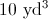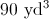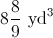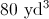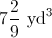Explanation:

Divide each dimension by 3 to convert feet to yards, then multiply the three dimensions together: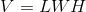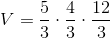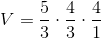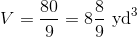### Example Question #6 : Prisms

Which is the greater quantity?

(A) The volume of a rectangular solid ten inches by twenty inches by fifteen inches

(B) The volume of a cube with sidelength sixteen inches

(A) and (B) are equal

(A) is greater

It is impossible to determine which is greater from the information given

(B) is greater

(B) is greater

Explanation:

The volume of a rectangular solid ten inches by twenty inches by fifteen inches is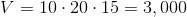cubic inches.

The volume of a cube with sidelength 13 inches is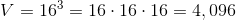cubic inches.

This makes (B) greater